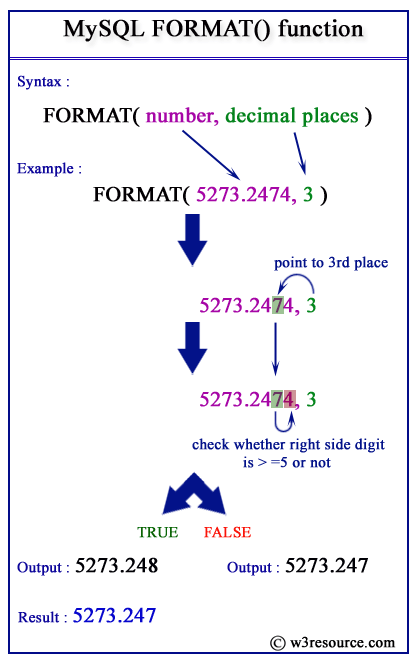# MySQL FORMAT() function

## FORMAT() function

MySQL FORMAT() converts a number to a format like ‘#,###,###.##’ which is rounded upto the number of decimal places specified (in the second argument) and returns the result as a string. There is no decimal point if the decimal place is defined as 0.

Syntax:

```FORMAT (N, D)
```

Arguments:

Name Description
N A number which may be an integer, a decimal or a double.
D An integer which specifies up to how many decimal places the return value is going to contain.

MySQL Version: 5.6

Video Presentation:

Pictorial Presentation:Example : MySQL FORMAT() function

The following MySQL statement calculates up to 3 decimal places of 12324.2573, so it returns 12,324.257.

Code:

``````SELECT FORMAT(12324.2573,3);
```
```

Sample Output:

```mysql> SELECT FORMAT(12324.2573,3);
+----------------------+
| FORMAT(12324.2573,3) |
+----------------------+
| 12,324.257           |
+----------------------+
1 row in set (0.02 sec)
```

Example of MySQL format() function using where clause

The following statement will return those books from the book_mast table, whose prices are more than 150. The price is returned up to 4 decimal places as specified in the argument.

Code:

``````SELECT book_name,FORMAT(book_price,4)
FROM book_mast
WHERE book_price>150;
```
```

Sample table: book_mast

Sample Output:

```mysql> SELECT book_name,FORMAT(book_price,4)
-> FROM book_mast
-> WHERE book_price>150;
+--------------------------------+----------------------+
| book_name                      | FORMAT(book_price,4) |
+--------------------------------+----------------------+
| Guide to Networking            | 200.0000             |
| Transfer  of Heat and Mass     | 250.0000             |
| Fundamentals of Thermodynamics | 225.0000             |
| Concepts in Health             | 180.0000             |
+--------------------------------+----------------------+
4 rows in set (0.05 sec)
```

PHP script:

``````<!doctype html>
<html lang="en">
<meta charset="utf-8">
<meta name="viewport" content="width=device-width, initial-scale=1">
<title>example-format-function - php mysql examples | w3resource</title>
<meta name="description" content="example-format-function - php mysql examples | w3resource">
<body>
<div class="container">
<div class="row">
<div class="col-md-12">
<h2>list of books and their prices if the book priced more than 150. Prices are formatted upto four places after decimal:</h2>
<table class='table table-bordered'>
<tr>
<th>Author's id</th><th>Prices</th>
</tr>
<?php
\$hostname="your_hostname";
\$db = "your_dbname";
foreach(\$dbh->query('SELECT book_name,FORMAT(book_price,4)
FROM book_mast
WHERE book_price>150') as \$row) {
echo "<tr>";
echo "<td>" . \$row['book_name'] . "</td>";
echo "<td>" . \$row['FORMAT(book_price,4)'] . "</td>";
echo "</tr>";
}
?>
</tbody></table>
</div>
</div>
</div>
</body>
</html>
```
```

View the example in browser

JSP script:

``````<%@page contentType="text/html" pageEncoding="UTF-8"%>
<%@ page import="java.sql.*" %>
<%@ page import="java.io.*" %>
<!DOCTYPE html>
<html>
<meta http-equiv="Content-Type" content="text/html; charset=UTF-8">
<title>example-format-function</title>
<body>
<%
try {
Class.forName("com.mysql.jdbc.Driver").newInstance();
String Host = "jdbc:mysql://localhost:3306/w3resour_bookinfo";
Connection connection = null;
Statement statement = null;
ResultSet rs = null;
connection = DriverManager.getConnection(Host, "root", "datasoft123");
statement = connection.createStatement();
String Data ="SELECT book_name,FORMAT(book_price,4) FROM book_mast WHERE book_price>150";
rs = statement.executeQuery(Data);
%>
<TABLE border="1">
<tr width="10" bgcolor="#9979">
<td>Author's id</td>
<td>Prices</td>
</tr>
<%
while (rs.next()) {
%>
<TR>
<TD><%=rs.getString("book_name")%></TD>
<TD><%=rs.getString("FORMAT(book_price,4)")%></TD>
</TR>
<%   }    %>
</table>
<%
rs.close();
statement.close();
connection.close();
} catch (Exception ex) {
out.println("Cant connect to database.");
}
%>
</body>
</html>
```
```

Online Practice Editor:

All String FunctionsPrevious: FIND_IN_SET
Next: HEX

﻿